# Partially balanced incomplete block design

PBIBD

A (symmetric) association scheme with $m$ classes on the $v$ symbols $\{1,...,v\}$ satisfies:

Two distinct symbols $x$ and $y$ are termed $i$th associates for exactly one $i\in\{1,...,m\}$; each symbol has exactly $n_i$ $i$th associates; and when two distinct symbols $x$ and $y$ are $i$th associates, the number of other symbols that are $j$th associates of $x$ and also $k$th associates of $y$ is $p^i_jk$, independent of the choice of the $i$th associates $x$ and $y$. The matrices $A_0,...,A_m$ of an $m$-class association scheme are defined as $A_0=I$, and for $1\leq \leq m$, $A_i$ is a $(0,1)$-matrix whose entry $(x,y)$ is $1$ exactly when $x$ and $y$ are $i$th associates.

Let $X$ be a $v$-set with a symmetric $m$-class association scheme defined on it. A partially balanced incomplete block design with $m$ associate classes (or $\mathrm{PBIBD}(m)$) is a block design based on $X$ with $b$ sets (the blocks), each of size $k$, and with each symbol appearing in $r$ blocks. Any two symbols that are $i$th associates appear together in $\lambda_i$ blocks of $\mathrm{PBIBD}(m)$. The numbers $v,b,r,k,\lambda_i$ ($1\leq i\leq m$) are the parameters of $\mathrm{PBIBD}(m)$. The notation $\mathrm{PBIBD}(v,k,\lambda_i)$ is also used. $N$ is used for the $v\times b$ $(0,1)$ incidence matrix of $\mathrm{PBIBD}(m)$.

Let $A_0,...,A_m$ be the matrices of an association scheme corresponding to a $\mathrm{PBIBD}(m)$. Then $NN^T=rI+\sum_i\lambda_iA_i$ and $JN=kJ$. Conversely, if $N$ is a $(0,1)$-matrix which satisfies these conditions and the $A_i$ are the matrices of an association scheme, then $N$ is the incidence matrix of a $\mathrm{PBIBD}(m)$.

It is easily verified that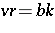, that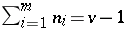, and that. A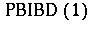is a balanced incomplete block design (a BIBD; cf. Block design); also, a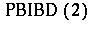in which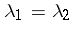is a BIBD.

There are six types ofs, [a3], based on the underlying types of association schemes:

1) group divisible;

2) triangular;

3) Latin-square-type;

4) cyclic;

5) partial-geometry-type; and

6) miscellaneous.

Partition the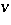-setinto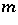groups each of size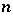. In a group-divisible association scheme the first associates are the symbols in the same group and the second associates are all the other symbols. The eigenvalues of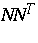are,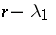and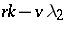, with multiplicities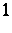,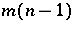, and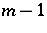, respectively. A group-divisible partially balanced incomplete block design is singular if; semi-regular if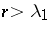,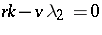; and regular ifand.

Let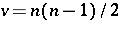,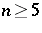, and arrange theelements of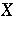in a symmetrical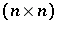-array with the diagonal entries blank. In the triangular association scheme, the first associates of a symbol are those in the same row or column of the array; all other symbols are second associates. The duals of triangulars are the residual designs of symmetric BIBDs with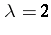. Triangular schemes and generalized triangular schemes are also known as Johnson schemes.

Let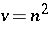and arrange thesymbols in an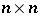array. Superimpose on this array a set of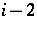mutually orthogonal Latin squares (see [a1] and also Latin square) of order. Let the first associates of any symbol be those in the same row or column of the array or be associated with the same symbols in one of the Latin squares. This is an-type association scheme. If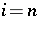, then the scheme is group divisible; if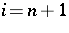, then all the symbols are first associates of each other.

Let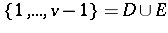. A non-group divisible association scheme defined onis cyclic if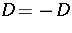and if the set of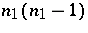differences of distinct elements of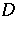has each element oftimes and each element of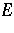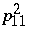times. The first associates ofare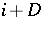.

In a partial-geometry-type association scheme, two symbols are first associates if they are incident with a line of the geometry and second associates if they are not incident with a line of the geometry.

See [a2], [a4], [a5] for further information.

How to Cite This Entry:
Partially balanced incomplete block design. Encyclopedia of Mathematics. URL: http://encyclopediaofmath.org/index.php?title=Partially_balanced_incomplete_block_design&oldid=17069
This article was adapted from an original article by C.J. Colbourn (originator), which appeared in Encyclopedia of Mathematics - ISBN 1402006098. See original article